##全国校区# 剧版“浪姐”口碑爆了！数据告诉你，《三十而已》凭什么这么戳中观众的心2020-07-28# CDA数据分析师 出品

【导读】

Python技术部分请直接看第四部分

Show me data，用数据说话

《乘风破浪的姐姐》已成为这个夏天最火爆的话题，前有国综"浪姐"高歌猛进，后有这部剧版"浪姐"《三十而已》让人眼前一亮。01女性角色不断霸屏

• 《致命女人》堪称复仇类肥皂剧顶级配置，
• 《大小谎言》将家庭里的谎言、爱与暴力演绎的淋漓尽致，
• 《了不起的麦瑟尔夫人》则让我们看到了上个世纪女性的辛酸和成长• 英剧《杀死伊芙》上演了一场正邪双方的猫鼠游戏
• 《我的天才女友》讲述了发生在意大利那不勒斯小城一段跨越漫长岁月的友谊
• 日剧《东京女子图鉴》和韩剧《请输入搜索词:WWW》也都将焦点剧集在都市女性的日常生活和职场中。02《三十而已》怎么突然火了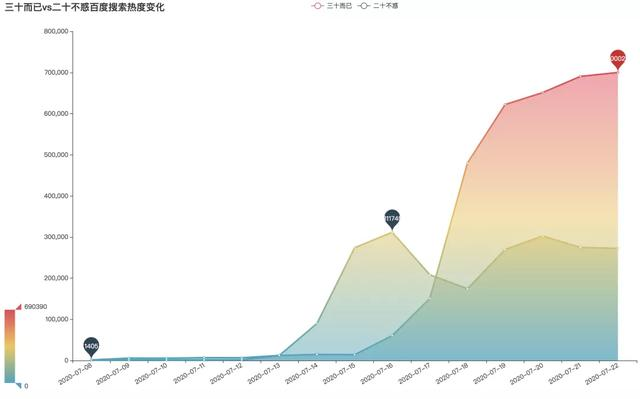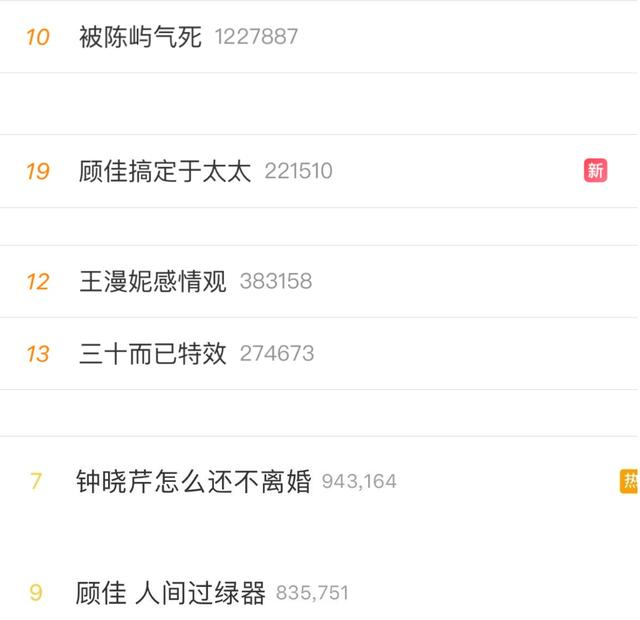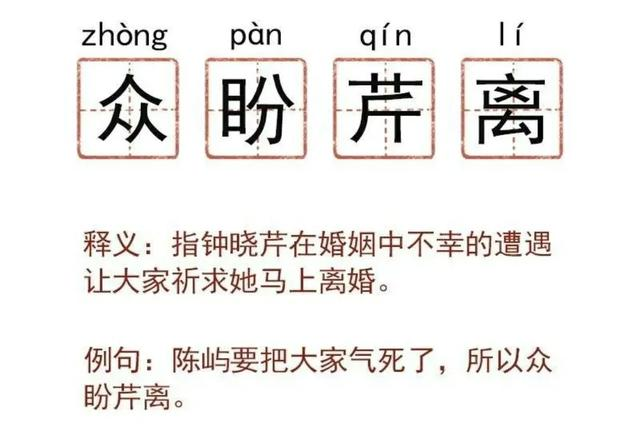03《三十而已》中是什么最戳中观众的心？

1豆瓣数据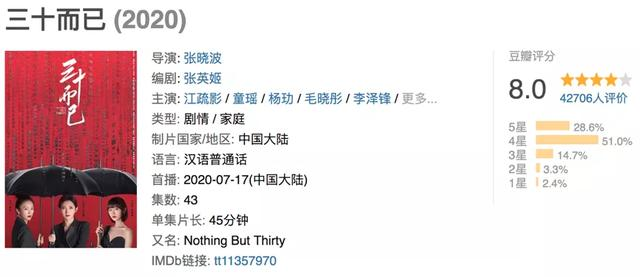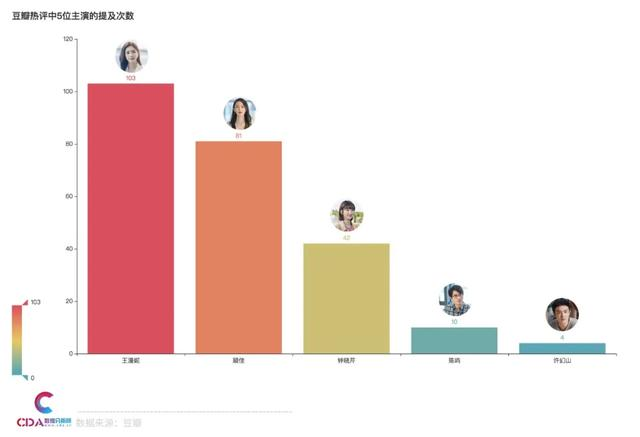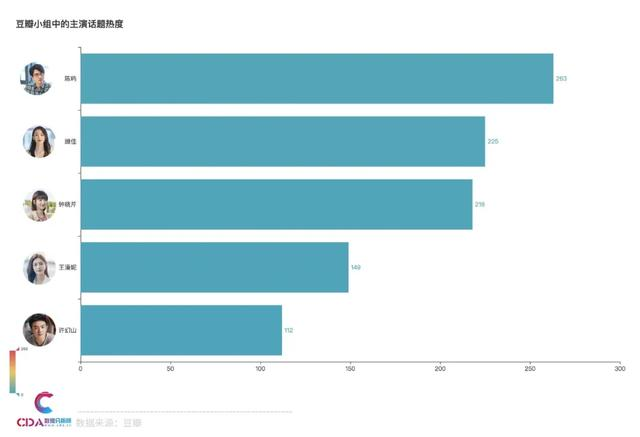2腾讯视频弹幕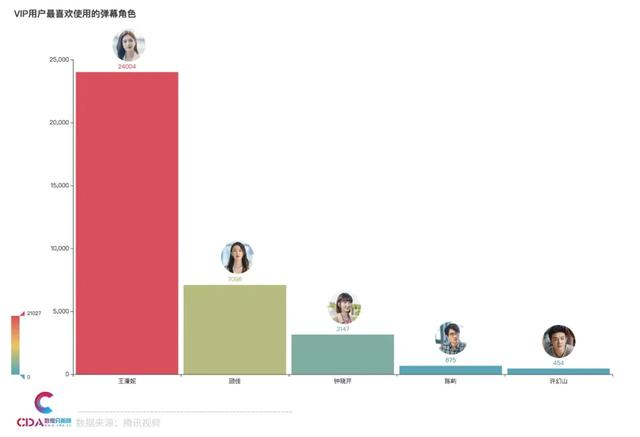04如何用Python获取弹幕数据

1. 弹幕数据获取和数据读入
2. 数据预处理
3. 数据可视化分析

1 数据读入

```# 导入库
import os
import jieba
import numpy as np
import pandas as pd

from pyecharts.charts import Bar, Pie, Line, WordCloud, Page
from pyecharts import options as opts
from pyecharts.globals import SymbolType

import stylecloud
from IPython.display import Image # 用于在jupyter lab中显示本地图```

```# 读入数据
data_list = os.listdir('../data/')

df_all = pd.DataFrame()

for i in data_list:
# 判断
if i.split('.')[-1] == 'csv':
df_one = pd.read_csv(f'../data/{i}', engine='python', encoding='utf-8', index_col=0)
df_all = df_all.append(df_one, ignore_index=False)

df_all.info() ```
```pandas.core.frame.dataframe'>
Int64Index: 271049 entries, 0 to 17637
Data columns (total 7 columns):
#   Column      Non-Null Count   Dtype
---  ------      --------------   -----
0   episodes    271049 non-null  int64
1   comment_id  271049 non-null  int64
2   oper_name   139035 non-null  object
3   vip_degree  271049 non-null  int64
4   content     271049 non-null  object
5   time_point  271049 non-null  int64
6   up_count    271049 non-null  int64
dtypes: int64(5), object(2)
memory usage: 16.5+ MBpandas.core.frame.dataframe'>```

```豆瓣数据# 提取数据
pattern = r'(王漫妮\s*|钟晓芹\s*|顾佳\s*|陈屿\s*|许幻山\s*|飒飒\s*|浪浪\s*):.*'
df_all['danmu_role'] = df_all['content'].str.extract(pattern).str.strip()

# 定义函数
def transform_name(x):
if x=='王漫妮' or x=='顾佳' or x=='钟晓芹' or x=='陈屿' or x=='许幻山' or x=='飒飒' or x=='浪浪':
return 'VIP用户'
elif x=='NaN':
return '未知用户'
else:
return '普通用户'

df_all['danmu_level'] = df_all['danmu_role'].apply(transform_name)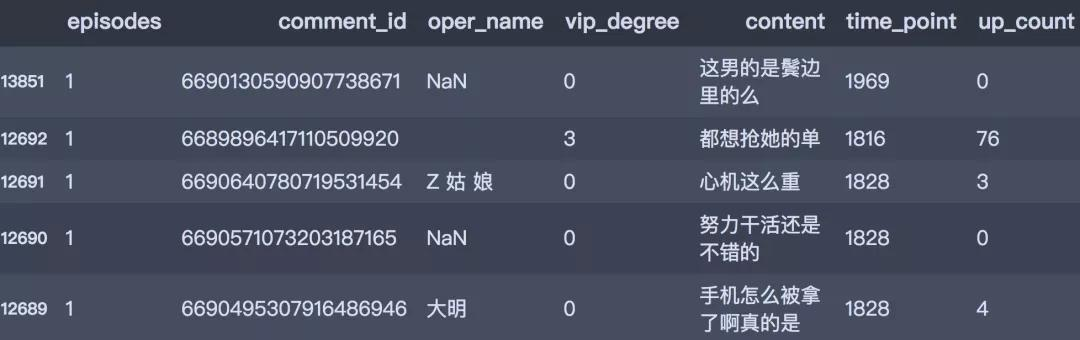2 数据预处理

```豆瓣数据# 提取数据
pattern = r'(王漫妮\s*|钟晓芹\s*|顾佳\s*|陈屿\s*|许幻山\s*|飒飒\s*|浪浪\s*):.*'
df_all['danmu_role'] = df_all['content'].str.extract(pattern).str.strip()

# 定义函数
def transform_name(x):
if x=='王漫妮' or x=='顾佳' or x=='钟晓芹' or x=='陈屿' or x=='许幻山' or x=='飒飒' or x=='浪浪':
return 'VIP用户'
elif x=='NaN':
return '未知用户'
else:
return '普通用户'

df_all['danmu_level'] = df_all['danmu_role'].apply(transform_name)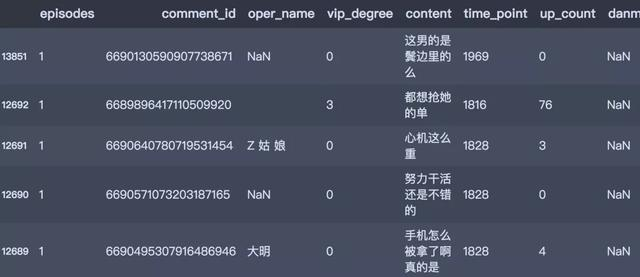# 弹幕发送人群等级分布

```level_num = df_all['danmu_level'].value_counts()

data_pair =  [list(z) for z in zip(level_num.index.tolist(), level_num.values.tolist())]

# 绘制饼图
pie1 = Pie(init_opts=opts.InitOpts(width='1350px', height='750px'))
pie1.set_global_opts(title_opts=opts.TitleOpts(title='弹幕发送人群等级分布'),
legend_opts=opts.LegendOpts(orient='vertical', pos_top='15%', pos_left='2%'))
pie1.set_series_opts(label_opts=opts.LabelOpts(formatter="{b}:{d}%"))
pie1.set_colors(['#6FB27C', '#FFAF34'])
pie1.render() ```

# 用户最喜欢使用的弹幕角色？

```role_num = df_all['danmu_role'].value_counts()
role_num.drop(['飒飒', '浪浪'], inplace=True)

# 柱形图
bar1 = Bar(init_opts=opts.InitOpts(width='1350px', height='750px'))
bar1.set_global_opts(title_opts=opts.TitleOpts(title="VIP用户最喜欢使用的弹幕角色"),
visualmap_opts=opts.VisualMapOpts(max_=21027),
)
bar1.render() ```

# 弹幕角色-王漫妮 词云图

```content_series1 = df_all[df_all.content.str.replace(pattern, '').str.contains('漫妮|疏影')]['content']
text1 = get_cut_words(content_series1)

# 绘制词云图
stylecloud.gen_stylecloud(text=' '.join(text1), max_words=1000,
collocations=False,
font_path=r'‪C:\Windows\Fonts\msyh.ttc',
icon_name='fas fa-heart',
size=653,
output_name='./html/弹幕角色王漫妮-词云图.png')```OK